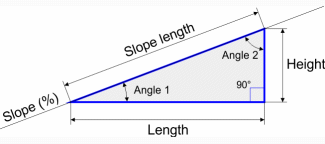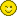# Calculate a slope, a gradient, a tilt or a pitch

## Calculating a Slope from the Length and Height

Calculating a slope using the width and height to find the percentage, angle or length of a slope (the hypotenuse*) is often useful in many areas – especially in the construction industry like stairs or roofs.

## Definition of a Slope

The slope corresponds to the inclination of a surface or a line in relation to the horizontal. It can be measured as an angle in degrees, radians or gradians or as a percentage (the width-to-height ratio multiplied by 100).

You can obtain all the necessary values for the pitch of a roof, a drainage slope, a steep path, an access ramp, etc..

## Calculating Slope

 Length: Height: Units: inch foot yard mile mm cm dm m dm hm km Decimal places: 0 1 2 3 4 5 6 7 8 9 10 Slope: 75,00 % Slope length: 5,00 m Angle 1: 36,87 ° Angle 2: 53,13 ° Perimeter of the triangle: 12,00 m Surface of the triangle: 6,00 m2* Hypotenuse: A wholly barbarian term which means the "length of the third side" or "slope length".## Formula for Calculating the Slope

The length of a slope is calculated using the Pythagorean Theorem. This theorem is done by multiplying the square's square root by the sum of the squared width and the squared height.

So: Square root ((width x width) + (height x height)) = Slope length

## An inclination of 100%

It should be noted that a slope of 100% is not vertical, but it is equivalent to having an identical height and width, which results in a 45° angle of inclination. For example: a mountain path with a 100% gradient would rise 100 meters for every 100 meters it goes forward. The distance along this slope would be 141.4 meters.

## Ramps for the Disabled

The slopes for a ramp for disabled access should be:

• maximum 12% for a length of less than 50 cm
• maximum 8% for length of less than 8 m
• between 4% and 5% for a maximum length of 10 m

Landings must be put in installed every 10 m maximum and have a minimum length of 1.40 m.

The gradient of the width, known as the cross slope, should preferably be zero, and in all cases less than 2%. The ramp width must be at least 1.40 m. It can be reduced to 1.20 m if there is no wall on one side or the other.

The gradient of the width, also known as the cross slope, should preferably be zero and should never exceed 2%. The ramp's width must be at least 1.40 m. It can be reduced to 1.20 m if one side is not attached to a wall.

## Average Inclination and Elevation of a Path

It is quite possible to calculate the average inclination of a path using the values given by the horizontal distance (run) and elevation (rise) reached at the end of the incline. If the path is winds, the distance traveled will be greater than the length of the slope.

## Drawing a Right Angle

The length of the slope corresponds to the diagonal of a right-angle triangle. You can also use this measurement to calculate the diagonal in order to draw a right angle, a technique widely used in the construction industry. Usually the values used are: 3 meters, 4 meters and 5 meters to obtain a right angle. Unfortunately, it is not always possible to use such measurements. Substituting the values of 3 meters and 4 meters with the values taken at the site and the result of the length of the slope (used as the diagonal), will allow you to correctly draw a right angle.